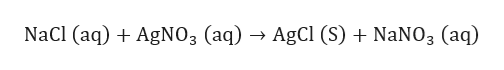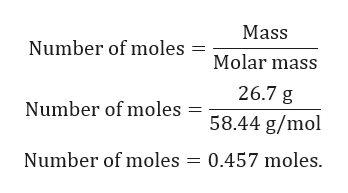For the following reaction, 26.7 grams of sodium chloride are allowed to react with 65.4 grams of silver nitrate . sodium chloride(aq) + silver nitrate(aq) silver chloride(s) + sodium nitrate(aq)What is the maximum amount of silver chloride that can be formed? What is the FORMULA for the limiting reagent?What amount of the excess reagent remains after the reaction is complete?

Question

For the following reaction, 26.7 grams of sodium chloride are allowed to react with 65.4 grams of silver nitrate .

sodium chloride(aq) + silver nitrate(aq) silver chloride(s) + sodium nitrate(aq)

What is the maximum amount of silver chloride that can be formed?

 What is the FORMULA for the limiting reagent?

What amount of the excess reagent remains after the reaction is complete?

Step 1

Given:

Mass of NaCl = 26.7 g.

Mass of AgNO3 = 65.4 g.

Step 2

Balanced chemical reaction is given by:help_outlineImage TranscriptioncloseNaCl (aq) AgNO3 (aq) AgCl (S) NaNO3 (aq) fullscreen
Step 3

Calculating number of ...help_outlineImage TranscriptioncloseMass Number of moles = Molar mass 26.7 g Number of moles 58.44 g/mol Number of moles 0.457 moles. fullscreen

Want to see the full answer?

See Solution

Want to see this answer and more?

Our solutions are written by experts, many with advanced degrees, and available 24/7

See Solution
Tagged in

General Chemistry